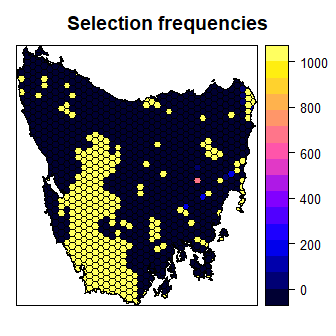# Tasmania Tutorial

## Introduction

The aim of this tutorial is to provide a worked example of how vector-based data can be used to develop conservation prioritizations using the prioritizr R package. It is also written for conservation planners that have used the Marxan decision support tool (Ball et al. 2009) and are interested in applying the prioritizr R package to their own work. The data set used in this tutorial was obtained from the “Introduction to Marxan” course. This data was originally a subset of a larger spatial prioritization project performed under contract to Australia’s Department of Environment and Water Resources (Klein et al. 2007).

Please note that this tutorial uses data from the prioritizrdata R, so ensure that it is installed before trying out the code yourself.

## Exploring the data

This data set contains two items. First, a spatial planning unit layer that has an attribute table which contains three columns: integer unique identifiers (“id”), unimproved land values (“cost”), and their existing level of protection (“status”). Units with 50 % or more of their area contained in protected areas are associated with a status of 2, otherwise they are associated with a value of 0. If you are familiar with the Marxan decision support tool, then you will notice that these columns are formatted in a similar manner to the input data for Marxan. For Marxan input data, planning units must be described in a table containing one row for each planning unit with a unique identifier and the corresponding cost.

The second item in this data set is the raster-based feature data. Specifically, the feature data is expressed as a stack of rasters (termed a RasterStack object). Here each layer in the stack represents the distribution of a different vegetation class in Tasmania, Australia. There are 62 vegetation classes in total. For a given layer, pixel values indicate the presence (value of 1) or absence (value of 0) of the vegetation class in an area.

First, load the required packages and the data.

# load packages
library(prioritizrdata)
library(prioritizr)

# load planning unit data
data(tas_pu)

# load conservation feature data
data(tas_features)

Now, let’s have a look at the planning unit data.

# print planning unit data
print(tas_pu)
## class       : SpatialPolygonsDataFrame
## features    : 1130
## extent      : 1080623, 1399989, -4840595, -4497092  (xmin, xmax, ymin, ymax)
## coord. ref. : +proj=aea +lat_1=-18 +lat_2=-36 +lat_0=0 +lon_0=132 +x_0=0 +y_0=0 +ellps=GRS80 +units=m +no_defs
## variables   : 5
## names       :   id,              cost, status, locked_in, locked_out
## min values  :    1, 0.192488262910798,      0,         0,          0
## max values  : 1130,  61.9272727272727,      2,         1,          0
# plot map of planning unit costs
spplot(tas_pu, zcol = "cost", names.attr = "Cost",
main = "Planning unit costs")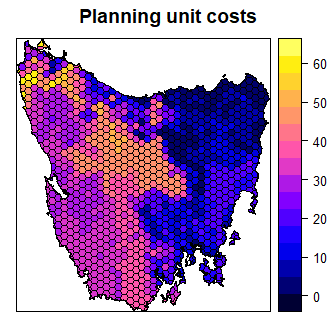Next, let examine the feature data. Here we will only plot the first four features as an example. The pixel values denote the presence or absence of each feature within the extent of the study area.

# print planning unit data
print(tas_features)
## class       : RasterStack
## dimensions  : 343, 320, 109760, 62  (nrow, ncol, ncell, nlayers)
## resolution  : 1000, 1000  (x, y)
## extent      : 1080496, 1400496, -4841217, -4498217  (xmin, xmax, ymin, ymax)
## coord. ref. : +proj=aea +lat_1=-18 +lat_2=-36 +lat_0=0 +lon_0=132 +x_0=0 +y_0=0 +ellps=GRS80 +units=m +no_defs
## names       : layer.1, layer.2, layer.3, layer.4, layer.5, layer.6, layer.7, layer.8, layer.9, layer.10, layer.11, layer.12, layer.13, layer.14, layer.15, ...
## min values  :       0,       0,       0,       0,       0,       0,       0,       0,       0,        0,        0,        0,        0,        0,        0, ...
## max values  :       1,       1,       1,       1,       1,       1,       1,       1,       1,        1,        1,        1,        1,        1,        1, ...
# plot map of the first four vegetation classes
plot(tas_features[[1:4]], main = paste("Feature", 1:4))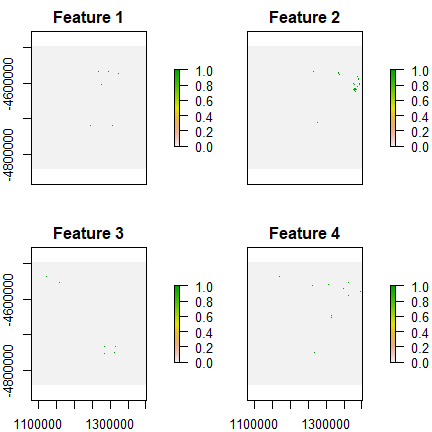The planning units in this example are a spatial polygon layer—not a raster—and so there can be considerable flexibility in the shape of the planning units. That said, there is a trade-off with the efficiency of data pre-processing in vector-based planning unit data compared to raster-based planning unit data. Vector-based planning unit data generally require more time to complete pre-processing computations (e.g. overlaying the planning unit data with the feature data, or generating boundary data). As a consequence, we generally recommend using raster-based planning unit data where possible to reduce processing time—but note that this is not possible when not all planning units are equal-sized squares. Another strategy is to complete the pre-processing in other software environments (e.g. ArcGIS) and use the pre-processed data directly with the prioritizr package.

## Marxan problem formulation

Here, we will provide an example of using the marxan_problem function to build and solve a typical Marxan conservation planning problem. Then we will show how this same problem can be built and solved using the fully customizable problem function as a comparison.

The data set used in this example follows many of the conventions used by the Marxan decision support tool. As a consequence, it is not too difficult to format the data for use with the marxan_problem function. The marxan_problem function is essentially a wrapper to the problem function. This means that when we solve problems created using the marxan_problem function, we will solve them using exact algorithms and not the simulated annealing algorithm used by Marxan.

All problem objects formulated with marxan_problem use the minimum set objective. Targets can be either relative or absolute, and planning units can be specified for masking in or out using the locked_in and locked_out arguments. To favor clumped solutions, use the penalty argument to impose a penalty on solutions with high boundary lengths (equivalent to the Boundary Length Modifier (BLM) used in Marxan), and the edge_factor argument to scale the penalty for edges that do not have neighboring planning units, such as the coastline. For simplicity we set all of the targets at the same level, 17 %, to reflect the Aichi biodiversity target to “safeguard” at least 17% of terrestrial ecosystems by 2020. For example, to prioritize planning units in Tasmania that meet the 17 % representation target at the least cost.

First, we will convert the vector-based planning unit data and raster-based feature data into the tabular formats required by the marxan_problem function. These formats are very similar to the formats used by Marxan.

# create table with planning unit data
pu_data <- tas_pu@data

# print first six rows
head(pu_data)
##   id     cost status locked_in locked_out
## 2  1 60.24638      0     FALSE      FALSE
## 3  2 19.86301      0     FALSE      FALSE
## 4  3 59.68051      0     FALSE      FALSE
## 5  4 32.41614      0     FALSE      FALSE
## 6  5 26.17706      0     FALSE      FALSE
## 7  6 51.26218      0     FALSE      FALSE
# create table with the feature identifiers, names, and targets
spec_data <- data.frame(id = seq_len(nlayers(tas_features)),
name = paste0("veg", seq_len(nlayers(tas_features))),
prop = 0.17)

# print first six rows
head(spec_data)
##   id name prop
## 1  1 veg1 0.17
## 2  2 veg2 0.17
## 3  3 veg3 0.17
## 4  4 veg4 0.17
## 5  5 veg5 0.17
## 6  6 veg6 0.17
# create table with the planning unit vs. feature data
puvspr_data <- rij_matrix(tas_pu, tas_features)
puvspr_data <- as(puvspr_data, "dgTMatrix")
puvspr_data <- data.frame(pu = puvspr_data@j + 1, species = puvspr_data@i + 1,
amount = puvspr_data@x)

# print first six rows
head(puvspr_data)
##   pu species     amount
## 1  3      54 0.02777778
## 2  3      59 0.33333333
## 3  5      30 0.05000000
## 4  6      27 0.03225806
## 5  6      35 0.03225806
## 6  7      12 0.01587302
# create table with the boundary data
bound_data <- boundary_matrix(tas_pu)
bound_data <- as(bound_data, "dgTMatrix")
bound_data <- data.frame(id1 = bound_data@i + 1, id2 = bound_data@j + 1,
boundary = bound_data@x)
# print first six rows
head(bound_data)
##   id1 id2  boundary
## 1   1   1 10259.010
## 2   3   1  3899.140
## 3   6   1   153.133
## 4   2   2 23878.508
## 5   4   2  3212.811
## 6   7   2  4725.951

Now that we have converted the data to tabular format, we can use the marxan_problem function to create a conservation planning problem.

# create problem
p1 <- marxan_problem(pu_data, spec_data, puvspr_data, bound_data,
blm = 0.0005)

# print problem
print(p1)
## Conservation Problem
##   planning units: data.frame (1130 units)
##   cost:           min: 0.19249, max: 61.92727
##   features:       veg1, veg2, veg3, ... (62 features)
##   objective:      Minimum set objective
##   targets:        Relative targets [targets (min: 0.17, max: 0.17)]
##   decisions:      default
##   constraints:    <Locked in planning units [257 locked units]>
##   penalties:      <Boundary penalties [edge factor (min: 1, max: 1), penalty (5e-04), zones]>
##   portfolio:      default
##   solver:         default

Next, we can solve the problem. The prioritizr R package supports three different exact algorithm software packages: gurobi, Rsymphony, and lpsymphony. There are costs and benefits associated with each of these different solvers, but each software should return similar results. Note that you will need at least one of these package installed on your system to solve problems. We recommend using the gurobi solver if possible, and have used this solver when building this tutorial. After solving the problem, we will calculate some statistics to describe the solution. Note that the planning unit selections are stored in the “solution_1” column of the solution object.

# solve problem
s1 <- solve(p1)
## Optimize a model with 6358 rows, 4278 columns and 14155 nonzeros
## Variable types: 0 continuous, 4278 integer (4278 binary)
## Coefficient statistics:
##   Matrix range     [1e-02, 1e+00]
##   Objective range  [5e-02, 2e+02]
##   Bounds range     [1e+00, 1e+00]
##   RHS range        [3e-03, 3e+00]
## Found heuristic solution: objective 30514.663295
## Found heuristic solution: objective 12420.567371
## Presolve removed 1797 rows and 1172 columns
## Presolve time: 0.03s
## Presolved: 4561 rows, 3106 columns, 9983 nonzeros
## Found heuristic solution: objective 12582.647264
## Found heuristic solution: objective 11565.031533
## Variable types: 0 continuous, 3106 integer (3106 binary)
## Presolved: 4561 rows, 3106 columns, 9983 nonzeros
##
##
## Root relaxation: objective 1.070324e+04, 739 iterations, 0.04 seconds
##
##     Nodes    |    Current Node    |     Objective Bounds      |     Work
##  Expl Unexpl |  Obj  Depth IntInf | Incumbent    BestBd   Gap | It/Node Time
##
##      0     0 10703.2364    0  100 11565.0315 10703.2364  7.45%     -    0s
##
## Explored 1 nodes (739 simplex iterations) in 0.10 seconds
## Thread count was 1 (of 8 available processors)
##
## Solution count 4: 11565 12417.3 12582.6 29785.4
##
## Optimal solution found (tolerance 1.00e-01)
## Best objective 1.156503153309e+04, best bound 1.070323643666e+04, gap 7.4517%
# print first six rows of solution object
head(s1)
##   id     cost status locked_in locked_out solution_1
## 2  1 60.24638      0     FALSE      FALSE          0
## 3  2 19.86301      0     FALSE      FALSE          0
## 4  3 59.68051      0     FALSE      FALSE          0
## 5  4 32.41614      0     FALSE      FALSE          0
## 6  5 26.17706      0     FALSE      FALSE          0
## 7  6 51.26218      0     FALSE      FALSE          0
# count number of planning units in solution
sum(s1$solution_1) ##  316 # proportion of planning units in solution mean(s1$solution_1)
##  0.279646
# calculate feature representation
r1 <- feature_representation(p1, s1[, "solution_1", drop = FALSE])
print(r1)
## # A tibble: 62 x 3
##    feature absolute_held relative_held
##    <chr>           <dbl>         <dbl>
##  1 veg1           0.107          0.176
##  2 veg2           0.563          0.172
##  3 veg3           0.0930         0.231
##  4 veg4           0.172          0.309
##  5 veg5           0.0775         0.191
##  6 veg6           0.0606         0.176
##  7 veg7           0.0462         0.191
##  8 veg8           0.185          0.248
##  9 veg9           1.46           0.242
## 10 veg10          0.0716         0.313
## # ... with 52 more rows
# visualize the solution by converting the solution to a spatial object
s1 <- SpatialPolygonsDataFrame(as(tas_pu, "SpatialPolygons"), data = s1)
s1$solution_1 <- factor(s1$solution_1)
spplot(s1, "solution_1", col.regions = c("grey90", "darkgreen"),
main = "marxan_problem solution")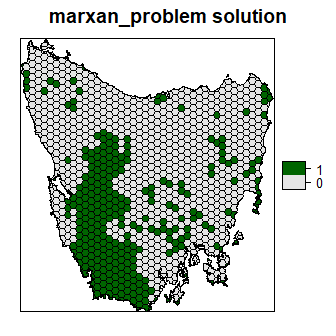## General problem formulation

Now we will formulate the exact same problem using the problem function and the spatially referenced data. We recommend using this approach instead of the marxan_problem because it is more verbose and you can specify exactly how the conservation planning problem should be formulated.

# build problem
p2 <- problem(tas_pu, tas_features, cost_column = "cost") %>%
add_boundary_penalties(penalty = 0.0005, edge_factor = 1)

# print the problem
print(p2)
## Conservation Problem
##   planning units: SpatialPolygonsDataFrame (1130 units)
##   cost:           min: 0.19249, max: 61.92727
##   features:       layer.1, layer.2, layer.3, ... (62 features)
##   objective:      Minimum set objective
##   targets:        Relative targets [targets (min: 0.17, max: 0.17)]
##   decisions:      Binary decision
##   constraints:    <Locked in planning units [257 locked units]>
##   penalties:      <Boundary penalties [edge factor (min: 1, max: 1), penalty (5e-04), zones]>
##   portfolio:      default
##   solver:         default
# solve problem
s2 <- solve(p2)
## Optimize a model with 6358 rows, 4278 columns and 14155 nonzeros
## Variable types: 0 continuous, 4278 integer (4278 binary)
## Coefficient statistics:
##   Matrix range     [1e-02, 1e+00]
##   Objective range  [5e-02, 2e+02]
##   Bounds range     [1e+00, 1e+00]
##   RHS range        [3e-03, 3e+00]
## Found heuristic solution: objective 30514.663295
## Found heuristic solution: objective 12420.567371
## Presolve removed 1797 rows and 1172 columns
## Presolve time: 0.03s
## Presolved: 4561 rows, 3106 columns, 9983 nonzeros
## Found heuristic solution: objective 12582.647264
## Found heuristic solution: objective 11565.031533
## Variable types: 0 continuous, 3106 integer (3106 binary)
## Presolved: 4561 rows, 3106 columns, 9983 nonzeros
##
##
## Root relaxation: objective 1.070324e+04, 739 iterations, 0.03 seconds
##
##     Nodes    |    Current Node    |     Objective Bounds      |     Work
##  Expl Unexpl |  Obj  Depth IntInf | Incumbent    BestBd   Gap | It/Node Time
##
##      0     0 10703.2364    0  100 11565.0315 10703.2364  7.45%     -    0s
##
## Explored 1 nodes (739 simplex iterations) in 0.07 seconds
## Thread count was 1 (of 8 available processors)
##
## Solution count 4: 11565 12417.3 12582.6 29785.4
##
## Optimal solution found (tolerance 1.00e-01)
## Best objective 1.156503153309e+04, best bound 1.070323643666e+04, gap 7.4517%
# calculate feature representation
r2 <- feature_representation(p2, s2[, "solution_1"])
print(r2)
## # A tibble: 62 x 3
##    feature  absolute_held relative_held
##    <chr>            <dbl>         <dbl>
##  1 layer.1         0.107          0.176
##  2 layer.2         0.563          0.172
##  3 layer.3         0.0930         0.231
##  4 layer.4         0.172          0.309
##  5 layer.5         0.0775         0.191
##  6 layer.6         0.0606         0.176
##  7 layer.7         0.0462         0.191
##  8 layer.8         0.185          0.248
##  9 layer.9         1.46           0.242
## 10 layer.10        0.0716         0.313
## # ... with 52 more rows
# visualize solution
s2$solution_1 <- factor(s2$solution_1)
spplot(s2, "solution_1", col.regions = c("grey90", "darkgreen"),
main = "problem solution")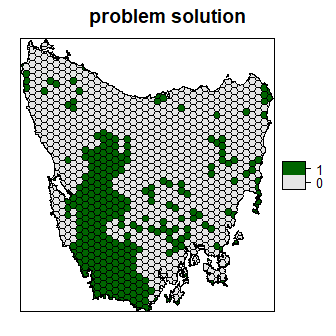## Selection frequencies

Similar to the Marxan decision support tool, we can generate a portfolio of solutions and compute the planning unit selection frequencies to understand their relative importance. This can be useful when trying to understand which planning units in the solution are the most irreplaceable. To do this, we will create a portfolio containing the top 1000 solutions nearest to optimality, and calculate the number of times that each planning unit was selected. Note that this requires the Gurobi software to be installed.

# create new problem with a portfolio added to it
p3 <- p2 %>%
add_gurobi_solver(gap = 0) %>%
add_pool_portfolio(method = 2, number_solutions = 1000)

# print problem
print(p3)
## Conservation Problem
##   planning units: SpatialPolygonsDataFrame (1130 units)
##   cost:           min: 0.19249, max: 61.92727
##   features:       layer.1, layer.2, layer.3, ... (62 features)
##   objective:      Minimum set objective
##   targets:        Relative targets [targets (min: 0.17, max: 0.17)]
##   decisions:      Binary decision
##   constraints:    <Locked in planning units [257 locked units]>
##   penalties:      <Boundary penalties [edge factor (min: 1, max: 1), penalty (5e-04), zones]>
##   portfolio:      Pool portfolio [method (2), number_solutions (1000)]
##   solver:         Gurobi [first_feasible (0), gap (0), presolve (2), threads (1), time_limit (2147483647), verbose (1)]
# generate solutions
s3 <- solve(p3)

# find column numbers with the solutions
solution_columns <- which(grepl("solution", names(s3)))

# calculate selection frequencies
s3$selection_frequencies <- rowSums(as.matrix(s3@data[, solution_columns])) # plot first four solutions in the portfolio s3$solution_1 <- factor(s3$solution_1) s3$solution_2 <- factor(s3$solution_2) s3$solution_3 <- factor(s3$solution_3) s3$solution_4 <- factor(s3$solution_4) spplot(s3, zcol = c("solution_1", "solution_2", "solution_3", "solution_4"), col.regions = c("grey90", "darkgreen"))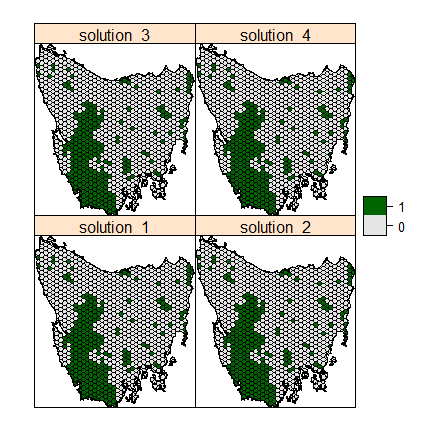# plot histogram of selection frequencies hist(s3$selection_frequencies, main = "Selection frequencies",
xlab = "Number of times units were selected")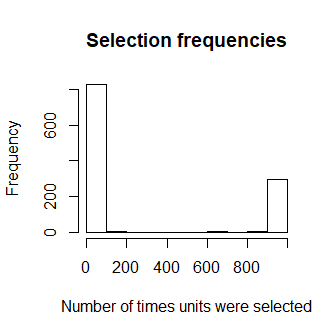# plot spatial distribution of the selection frequencies
spplot(s3, "selection_frequencies", main = "Selection frequencies")